# Python 字典类型3

``````dic = {'a': 1, 'b': 2, 'c': 3}
del dic['a']
print(dic)``````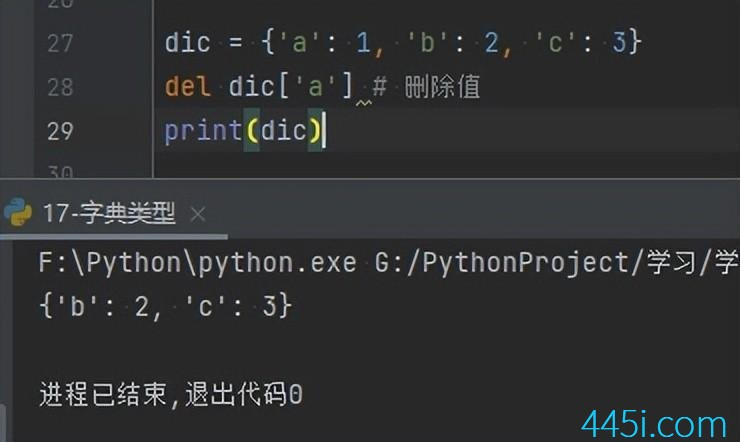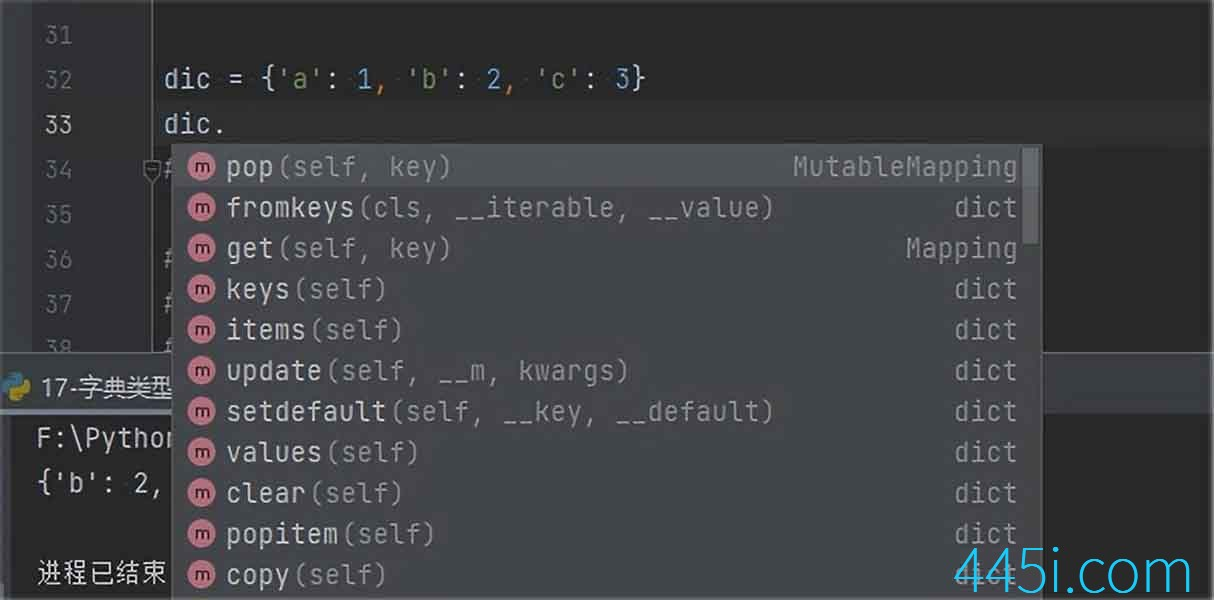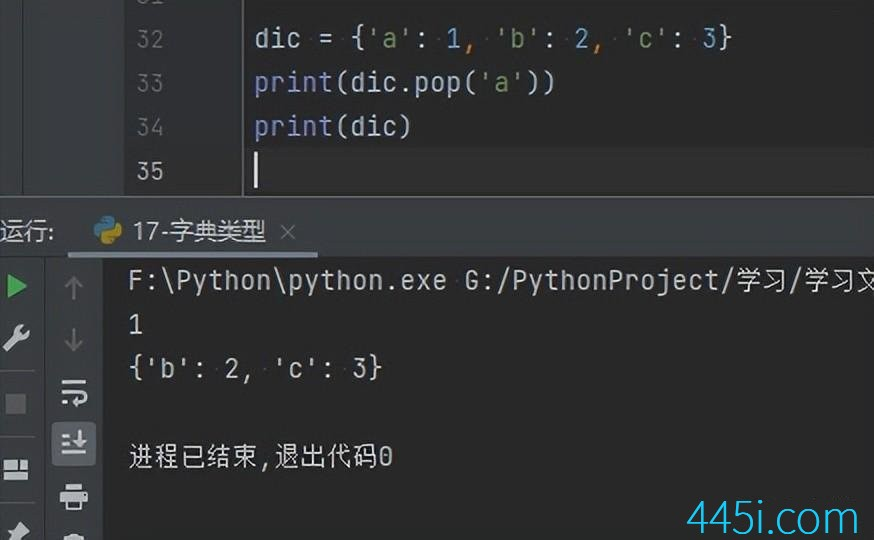``````dic = {'a': 1, 'b': 2, 'c': 3}
print(dic.popitem())``````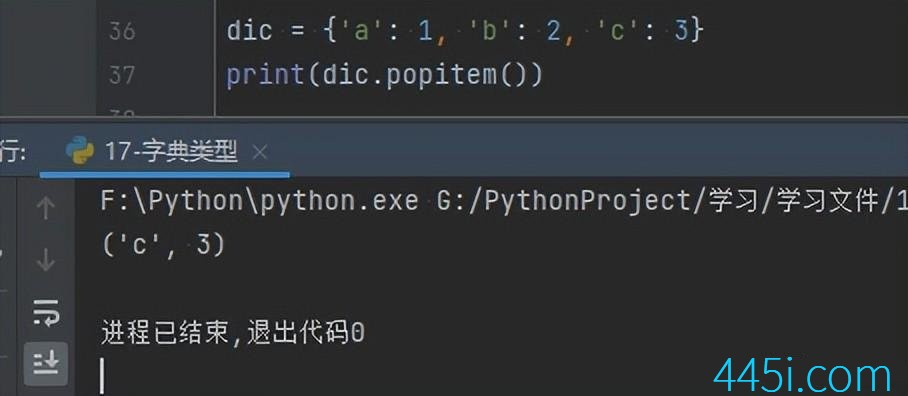``````dic = {'a': 1, 'b': 2, 'c': 3}
dic.clear()
print(dic)````````````dic = {'a': 1, 'b': 2, 'c': 3}
print(len(dic))````````````dic = {'aaa': 1, 2: 2, 3.2: 4, (7, 8, 9): 10}
print('1' in dic)``````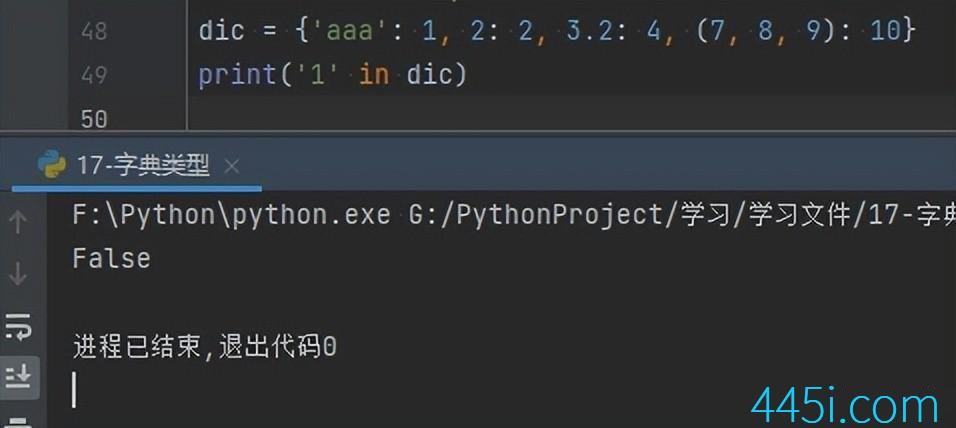``````dic = {'aaa': 1, 2: 2, 3.2: 4, (7, 8, 9): 10}
print(2 in dic)``````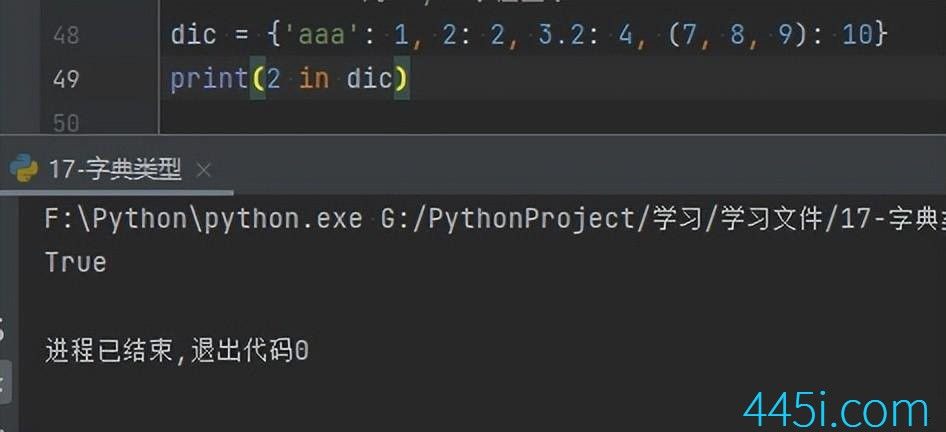### 觉得文章有用就打赏一下文章作者

#### 支付宝扫一扫打赏#### 微信扫一扫打赏